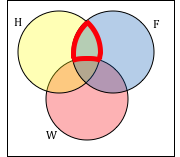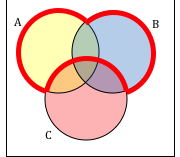## Set Theory Basics

### Learning Outcomes

• Describe memberships of sets, including the empty set, using proper notation, and decide whether given items are members and determine the cardinality of a given set
• Perform the operations of union, intersection, complement, and difference on sets using proper notation
• Describe the relations between sets regarding membership, equality, subset, and proper subset, using proper notation
• Be able to draw and interpret Venn diagrams of set relations and operations and use Venn diagrams to solve problems
• Recognize when set theory is applicable to real-life situations, solve real-life problems, and communicate real-life problems and solutions to others

It is natural for us to classify items into groups, or sets, and consider how those sets overlap with each other. We can use these sets understand relationships between groups, and to analyze survey data.

## Introduction to Set Theory

An art collector might own a collection of paintings, while a music lover might keep a collection of CDs. Any collection of items can form a set.

### Set

A set is a collection of distinct objects, called elements of the set

A set can be defined by describing the contents, or by listing the elements of the set, enclosed in curly brackets.

### Example

Some examples of sets defined by describing the contents:

1. The set of all even numbers
2. The set of all books written about travel to Chile

Some examples of sets defined by listing the elements of the set:

1. {1, 3, 9, 12}
2. {red, orange, yellow, green, blue, indigo, purple}

### Notation

Commonly, we will use a variable to represent a set, to make it easier to refer to that set later.

The symbol ∈ means “is an element of”.

A set that contains no elements, { }, is called the empty set and is notated ∅

### Example

Let A = {1, 2, 3, 4}

To notate that 2 is element of the set, we’d write 2 ∈ A

A set simply specifies the contents; order is not important. The set represented by {1, 2, 3} is equivalent to the set {3, 1, 2}.

### Subsets

Sometimes a collection might not contain all the elements of a set. For example, Chris owns three Madonna albums. While Chris’s collection is a set, we can also say it is a subset of the larger set of all Madonna albums.

### Subset

A subset of a set A is another set that contains only elements from the set A, but may not contain all the elements of A.

If B is a subset of A, we write B ⊆ A

A proper subset is a subset that is not identical to the original set—it excludes at least one element of the original set.

If B is a proper subset of A, we write BA

### Example

Consider these three sets:

A = the set of all even numbers
B = {2, 4, 6}
C = {2, 3, 4, 6}

Here BA since every element of B is also an even number, so is an element of A.

More formally, we could say BA since if x ∈ B, then x A.

It is also true that BC.

C is not a subset of A, since C contains an element, 3, that is not contained in A

### Example

Suppose a set contains the plays “Much Ado About Nothing,” “MacBeth,” and “A Midsummer’s Night Dream.” What is a larger set this might be a subset of?

### Try It

Consider the set $A = \{1, 3, 5\}$. Which of the following sets is $A$ a subset of?
$X = \{1, 3, 7, 5\}$
$Y = \{1, 3 \}$
$Z = \{1, m, n, 3, 5\}$

### Exercises

Given the set: A = {a, b, c, d}. List all of the subsets of A

Listing the sets is fine if you have only a few elements. However, if we were to list all of the subsets of a set containing many elements, it would be quite tedious. Instead, in the next example we will consider each element of the set separately.

### Example

In the previous example, there are four elements. For the first element, a, either it’s in the set or it’s not. Thus there are 2 choices for that first element. Similarly, there are two choices for b—either it’s in the set or it’s not. Using just those two elements, list all the possible subsets of the set {a,b}

Now let’s include c, just for fun. List all the possible subsets of the new set {a,b,c}.
Again, either c is included or it isn’t, which gives us two choices. The outcomes are {}, {a}, {b}, {c}, {a,b}, {a,c}, {b,c}, {a,b,c}. Note that there are $2^{3}=8$ subsets.

If you include four elements, there would be $2^{4}=16$ subsets. 15 of those subsets are proper, 1 subset, namely {a,b,c,d}, is not.

In general, if you have n elements in your set, then there are $2^{n}$ subsets and $2^{n}−1$ proper subsets.

## Union, Intersection, and Complement

Commonly, sets interact. For example, you and a new roommate decide to have a house party, and you both invite your circle of friends. At this party, two sets are being combined, though it might turn out that there are some friends that were in both sets.

### Union, Intersection, and Complement

The union of two sets contains all the elements contained in either set (or both sets). The union is notated A B. More formally, x A B if x A or x B (or both)

The intersection of two sets contains only the elements that are in both sets. The intersection is notated A B. More formally, x A B if x A and x B.

The complement of a set A contains everything that is not in the set A. The complement is notated A’, or Ac, or sometimes ~A.

A universal set is a set that contains all the elements we are interested in. This would have to be defined by the context.

A complement is relative to the universal set, so Ac contains all the elements in the universal set that are not in A.

### Example

1. If we were discussing searching for books, the universal set might be all the books in the library.
3. If you were working with sets of numbers, the universal set might be all whole numbers, all integers, or all real numbers

### Example

Suppose the universal set is U = all whole numbers from 1 to 9. If A = {1, 2, 4}, then Ac = {3, 5, 6, 7, 8, 9}.

### Example

Consider the sets:

A = {red, green, blue}
B = {red, yellow, orange}
C = {red, orange, yellow, green, blue, purple}

Find the following:

1. Find A B
2. Find A B
3. Find Ac C

### Try It

Notice that in the example above, it would be hard to just ask for Ac, since everything from the color fuchsia to puppies and peanut butter are included in the complement of the set. For this reason, complements are usually only used with intersections, or when we have a universal set in place.

As we saw earlier with the expression Ac  C, set operations can be grouped together. Grouping symbols can be used like they are with arithmetic – to force an order of operations.

### Example

Suppose H = {cat, dog, rabbit, mouse}, F = {dog, cow, duck, pig, rabbit}, and W = {duck, rabbit, deer, frog, mouse}

1. Find (H F) ⋃ W
2. Find H ⋂ (F W)
3. Find (H F)c W

### Venn Diagrams

To visualize the interaction of sets, John Venn in 1880 thought to use overlapping circles, building on a similar idea used by Leonhard Euler in the 18th century. These illustrations now called Venn Diagrams.

### Venn Diagram

A Venn diagram represents each set by a circle, usually drawn inside of a containing box representing the universal set. Overlapping areas indicate elements common to both sets.

Basic Venn diagrams can illustrate the interaction of two or three sets.

### Example

Create Venn diagrams to illustrate A B, A B, and Ac B

A B contains all elements in either set.

### Example

Use a Venn diagram to illustrate (H F)c W

### Example

Create an expression to represent the outlined part of the Venn diagram shown.### Try It

Create an expression to represent the outlined portion of the Venn diagram shown.## Cardinality

Often times we are interested in the number of items in a set or subset. This is called the cardinality of the set.

### Cardinality

The number of elements in a set is the cardinality of that set.

The cardinality of the set A is often notated as |A| or n(A)

### Exercises

Let A = {1, 2, 3, 4, 5, 6} and B = {2, 4, 6, 8}.

What is the cardinality of B? A B, A B?

### Exercises

What is the cardinality of P = the set of English names for the months of the year?

### Exercises

A survey asks 200 people “What beverage do you drink in the morning”, and offers choices:

• Tea only
• Coffee only
• Both coffee and tea

Suppose 20 report tea only, 80 report coffee only, 40 report both.   How many people drink tea in the morning? How many people drink neither tea or coffee?

### Example

A survey asks:   Which online services have you used in the last month:

• Have used both

The results show 40% of those surveyed have used Twitter, 70% have used Facebook, and 20% have used both. How many people have used neither Twitter or Facebook?

The previous example illustrated two important properties called cardinality properties:

### Cardinality properties

1. n(AB) = n(A) + n(B) – n(AB)
2. n(Ac) = n(U) – n(A)

Notice that the first property can also be written in an equivalent form by solving for the cardinality of the intersection:

n(AB) = n(A) + n(B) – n(AB)

### Example

Fifty students were surveyed, and asked if they were taking a social science (SS), humanities (HM) or a natural science (NS) course the next quarter.

21 were taking a SS course                  26 were taking a HM course

19 were taking a NS course                 9 were taking SS and HM

7 were taking SS and NS                     10 were taking HM and NS

3 were taking all three                         7 were taking none

How many students are only taking a SS course?

### Try It

One hundred fifty people were surveyed and asked if they believed in UFOs, ghosts, and Bigfoot.

43 believed in UFOs                           44 believed in ghosts

25 believed in Bigfoot                         10 believed in UFOs and ghosts

8 believed in ghosts and Bigfoot         5 believed in UFOs and Bigfoot

2 believed in all three

How many people surveyed believed in at least one of these things?